# Monthly Archives: January 2018

## Digging Deeper into … Decimals and Percentages (3rd – 6th)

Category : Uncategorized

For practical suggestions for families, and links to useful digital resources, to support children learning about this topic, please check out the following post: Dear Family, your Operation Maths Guide to Decimals and Percentages

In Operation Maths, decimals sits as a chapter of its own in third and fourth classes (allowing for specific time to focus on this new concept) and as a chapter combined with fractions and percentages in 5th and 6th classes. However, it is worth noting that the children would have informally encountered decimals since being introduced to euro and cent in first class. And, since decimals are inherently linked with both fractions and the place value system*, these topics, also, were encountered initially in first class, and thus the children’s understanding of decimals and percentages in the senior classes builds on their understanding of these related concepts. Indeed, in Operation Maths 5 and 6, the place value chapters include both whole and decimal numbers, since both are part of our base-ten system.

HINT: Read the related Operation Maths blog posts on fractions and place value at the links given.

### Representing decimals and percentages

In keeping with the CPA approach used throughout Operation Maths, the initial introductory activities concentrate on experiences with concrete materials (e.g. straws and money, as shown below) and pictorial activities (e.g. colouring in fractions of shapes, dividing shapes and completing number lines) before progressing to abstract questions, where the focus is primarily on numbers and/or digits.  Concrete and pictorial-based experiences also promote the development of number sense and visualisation skills, allowing the children to become more adept at converting between various forms and, ultimately, being able to order, compare and calculate with fractions, decimals and percentages more efficiently and accurately.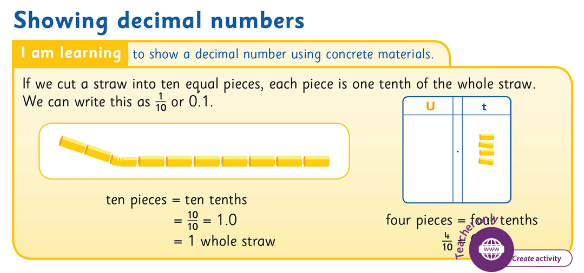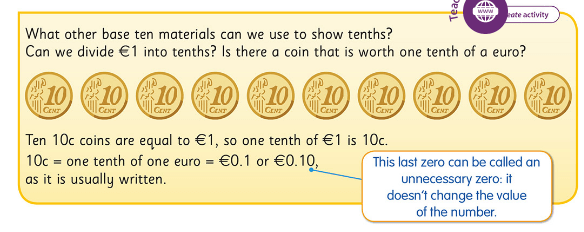In third class, the children encounter straws, place value discs, euro coins and blocks as examples of base-ten materials to represent hundreds, tens and units. Since, to represent one tenth, it is necessary to be able to fraction a unit, the only suitable base-materials to use are straws and ten cent coins (ie one tenth of a euro). Cutting up a straw into tenths really helps to demonstrate that one tenth is a very small part or bit of the whole. Operation Maths users can also use the Place Value eManipulative, accessible via Edco Learning to show various decimal numbers on the class IWB (see below).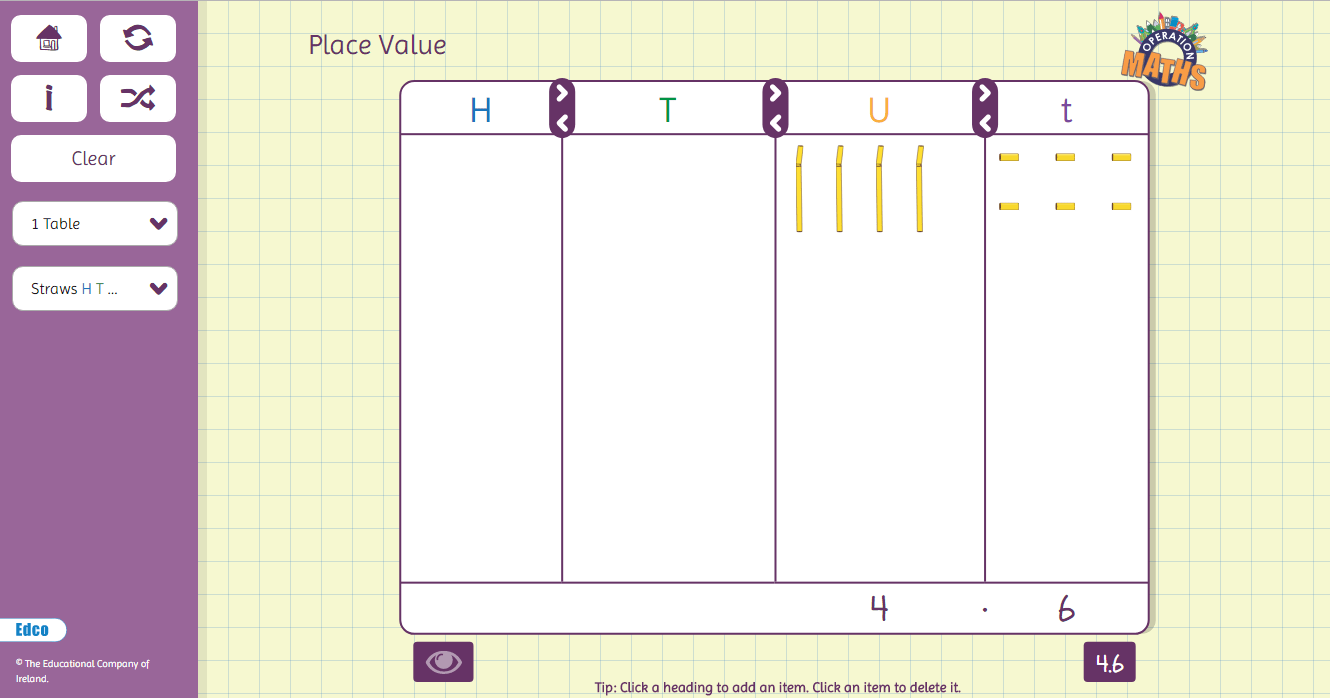In fourth class, with the introduction of the hundredth, the concrete materials become more limited, and one cent coins are used to represent hundredths of a euro (see below). Again, the Place Value eManipulative can also be used to represent hundredths; just select the Money HTU.th option from the drop down menu.NB: With rounding of prices to the nearest 5c, we are likely to see fewer 1c and 2c coins in circulation, although recognition and knowledge of 1c and 2c coins are still part of the curriculum. Therefore, it was decided to continue to include them throughout the Operation Maths series for both this reason and because they are valuable for teaching purposes, especially when teaching decimals, as shown above.

By fifth class, with the introduction of thousandths, the concrete possibilities have become even more limited; there is no coin to represent one thousandth of a euro because it is such a small and insignificant amount.  To this end, it it necessary to re-use base ten blocks, but with new values assigned to each block type (see below). It is very important that this is emphasized to the children,  and that they understand that the block that was previously used to represent a unit in 3rd and 4th class, is now being used to represent a thousandth in 5th and 6th class, simply because there are no other options!Types of traditional representations for decimal numbers that have deliberately not been used throughout the Operation Maths series are place value abaci and dot notation boards. As mentioned previously in the post on Place Value, tasks which just involve the children identifying the number of dots on a notation board, or the number of beads on a place value abacus have not been included as they are not good indicators of a child’s understanding; rather they are simply demonstrating their number knowledge of numbers and digits from 0–9.

With the introduction of percentages in fifth class, since percentages are directly related to hundredths, all of the concrete resources that can represent tenths and hundredths can be used again to represent a percentage. When exploring how percentages relate to fractions and decimals, multiple hundredths squares can be used to encourage the visualisation of the various fractions, as in done in the Operation Maths Discovery book for fifth class (see below).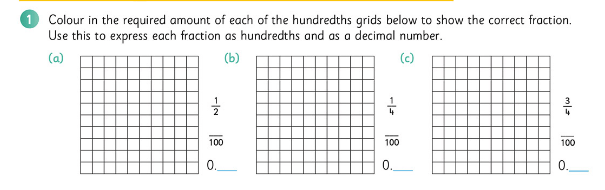### ‘Same value, different appearance’

Because of the close connections between decimals, percentages and fractions, it is very important from the beginning that decimals (or to be more exact, decimal fractions) and percentages (from their introduction in 5th class), are taught as connected concepts with fractions (e.g. 1/2 = 0.5 = 50%,  1/4 = 0.25 = 25%) and that the children are encouraged to recognise them as different forms of an equivalent value i.e. ‘same value, different appearance’. Being able to convert into equivalent forms becomes very important when it comes to ordering parts of a whole that are expressed in various forms (see below).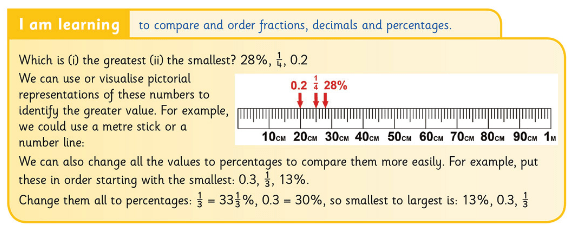Similarly, the children should be encouraged from the beginning to use both decimal language and fractional language when verbalising decimal notation, i.e. expressing 7.38 as ‘seven point three eight’ and also as ‘seven and thirty-eight hundredths’*. Using fractional language to read decimals reinforces the value of the digit(s) in the decimal place(s).

*However, when using decimal language, it is mathematically incorrect to say ‘seven point thirty-eight’, as the suffix -ty means tens.

Furthermore, the same decimal value can be written in various ways eg one-tenth can be written as 0.1, .1, 0.10, 0.100, etc. Many teachers often use only one form, usually 0.1, fearing that a variety of ways may confuse children. Conversely, using a variety of ways can actually help reinforce children’s understanding that all the above forms show one-tenth, with all forms (excluding .1) including unnecessary zeros.*
*Zeros can be necessary or unnecessary. In 30, the zero is necessary as without it, the value would be 3 units. In 0.3, the zero is unnecessary as without it, the value is still three-tenths.

### Calculations with decimals and percentages

Addition and subtraction involving decimals can often appear to be mastered, until the children start using decimals of differing lengths (sometimes referred to as ragged decimals), and subsequent errors at this stage can reveal gaps in the children’s understanding of the concept. Remind the children regularly to lay out calculations so that the decimal places and decimal points are in line, and also use concrete materials (e.g. €1, 10c and 1c coins shown earlier) to encourage them to visualise the component parts of the number and how these similar parts must be added or subtracted accordingly e.g. for 1.24 + 2.3,  ‘4 hundredths and zero hundredths equals 4 hundredths, 2 tenths and 3 tenths equals 5 tenths’ etc.

Similarly, multiplication with decimals can also reveal gaps in understanding e.g. 0.4 × 3 might be answered as 0.12; again, where possible use concrete materials (e.g. use the tenths of the straws) to encourage the children to visualise the numbers i.e. ‘4 tenths times 3  (or three groups of four tenths) is 4 tenths plus 4 tenths plus 4 tenths, which is 12 tenths’; if straws are used we can see how that is equal to one whole straw and 2 tenths i.e. 1.2. Therefore, if interpreting multiplication as “group(s) of”, it is important that the children appreciate that 0.5 x 3 can be thought of as 1/2 group of 3 or as 3 groups of 1/2, both of which equal 1 1/2; this is modeled below using the Operation Maths Bar Model eManipulative, accessible on edcolearning.ie.Another way to think about multiplications is as “rows of” and this concept of row leads very logically to the area model of multiplication. Base ten blocks are a very useful concrete material that can be used to represent multiplication with decimals via the area model, however it is important that the children appreciate that we are giving new values to each block ie each flat represents 1, the rods each represent 0.1/one tenth and the small cubes each represent 0.01/one hundredth. For more on how to use the area model of multiplications with decimals, please read on here: https://www.mathcoachscorner.com/2015/09/multiplying-decimals/The cartoon above clearly illustrates how fostering number sense is as important as teaching procedures:
2.95 is nearly 3
So 2.95 x 3.2 is roughly 3 x 3 which is 9.something
The decimal point therefore goes in after 9

When solving a problem involving percentages, it is generally more efficient to convert the percentage to an equivalent fraction or decimal, and then solve the problem using a fraction or decimal approach; the decimal approach is often the most efficient  to use in a situation where a calculator is available/allowed. When using a fraction approach the children should be encouraged to use bar models to represent the quantities involved (see below).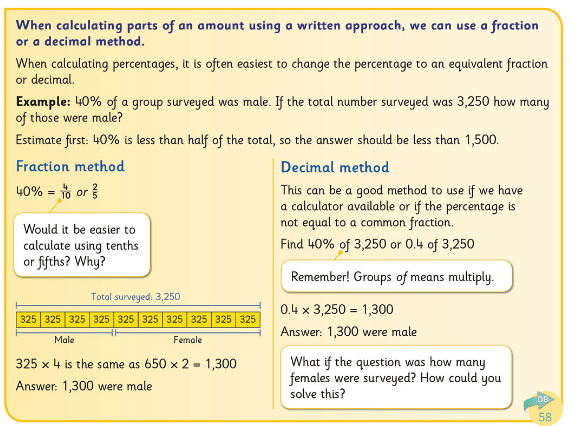All activities should emphasise understanding and not just a procedural approach.
While it is important that children in sixth class are shown ways to calculate with more complex numbers, it is vital that this is done in such a way that the children begin to understand the purpose of the approaches and how they work (see below). Being told to just ‘multiply by 100/1’ or ‘divide by the bottom, multiply by the top’, does little to enable the child to understand the concept better.### Incorrect assumptions & misconceptions

As discussed earlier, while fractions, decimals, percentages and place value understanding are inherently connected, children may apply whole number and fractional understanding to decimals and percentages in such a way as to make incorrect assumptions.

For example:

• A child may incorrectly assume that a number with more digits is bigger than a number with fewer digits, i.e. assuming incorrectly that 2.1 < 1.35
• In fractions, as the denominator increases the fraction parts themselves get smaller (e.g. 1/2 > 1/10). Therefore, a child may assume, also incorrectly, that a decimal number with more digits is smaller, e.g. 1.32 < 1.2.
• If 1/4 is greater than 1/8 , then a child may assume that 1.4 is greater than 1.8.
• A child may convert a decimal value directly to a percentage (and vice versa), without changing their value, e.g. incorrectly assuming 0.15 = 0.15%.

There can be many other commonly held misconceptions and errors, including a child incorrectly thinking that:

• Fractions, decimals and percentages are only parts of shapes and not numbers in their own right.
• Percentages don’t go above 100% and fractions are never greater than 1.
• A fraction such as 3/4 is only 3 groups of 1/4 without recognition that it can also be a 1/4  of 3; this is essential to understanding how a fraction can be converted into a decimal.
• To calculate 20% of an amount, you divide by 20 (since to calculate 10% you divide by 10).
• 0.2 equals a half or 12% is one twelfth, etc.

Again, many of these misconceptions and incorrect assumptions can be avoided by using a CPA approach to the teaching of this topic, with an emphasis on understanding rather than just doing.

• Dear Family, your Operation Maths Guide to Decimals and Percentages includes practical suggestions for supporting children, and links to a huge suite of digital resources, organised according to class level.
• Virtual Maths Manipulatives for Fractions, Decimals and Percentages: Lots of tools that can be used in many different ways to explore these concepts.
• Operation Maths users don’t forget to check out the extensive digital resources available for this topic on Edco Learning. These include Maths Around Us and Write, Hide, Show videos, Ready to go activities and Create Activities using the place value eManipulative, fractions eManipulative, 100 square eManipulative and bar modelling eManipulative. Operation Maths users in 3rd to 6th should check out the first page of the chapter in their Pupils Books for a quick synopsis of the suggested digital resources and then refer to their TRB for more detailed information.
• Place Value, Decimals and Percentages Manual from PDST
• NRICH: selection of problems, articles and games for decimals and percentages
• Check out this Pinterest Board for further ideas for decimals and percentages as well as fractions.
• Does the decimal point move? A one minute video which shows multiplying/dividing by 10/100 etc using the moving digits approach
• Watch this video showing a teacher revising tenths and introducing hundredths

## Digging Deeper into … Patterns and Sequences

This can be a difficult strand unit to track through the 1999 Primary Mathematics Curriculum; in junior and senior infants it is titled Extending patterns, in first and second classes it becomes Extending and using patterns, in third and fourth it is called Number patterns and sequences,  and in fifth and sixth classes it morphs into Rules and properties. However, it is always from the strand Algebra (check out the maths curriculum glance cards here for more detail) and a summary of the objectives reveals how pattern is at the heart of the strand unit at every class level:

Junior  Infants to Second Class > Algebra > Extending patterns >

• identify, copy and extend patterns (colour, shape, size, number)
• recognise patterns (including odd and even numbers;  predict subsequent numbers)
• explore and use patterns in addition facts (1st & 2nd)

Third & Fourth Class > Algebra > Number patterns and sequences >
• explore, recognise and record patterns in number
• explore, extend and describe (explain rule for) sequences
• use patterns as an aid in the memorisation of number facts

Fifth & Sixth Class > Algebra > Rules and properties >
• identify relationships and record verbal and simple symbolic rules for number patterns

### Different types of patterns

So then, are patterns and sequences the same thing? Actually, there are two main types of patterns:

• Repeating patterns: repetitions of symbols, shapes, numbers etc., that recur in a specific way.
• Increasing (growing) and decreasing (shrinking) patterns: An ordered set of shapes or numbers that are arranged according to a rule. Typically, sequence is also used to describe an increasing or decreasing pattern, particularly if it is a pattern of numbers.

### Repeating Patterns

A repeating pattern should have a clearly identifiable core, i.e. the shortest sequence that repeats. It is a good idea to use the terminology of “core” right from the infant classes so that the children understand what is being asked of them.Children can often copy patterns without even recognising or identifying the core. However, to become competent in accurately extending repeating patterns, it is vital to identify the core. Ways that the children can become more adept at this include verbalising the pattern out loud (“red, blue, yellow, red, blue, yellow, …”) and/or using concrete materials to model the pattern (see below); in this way it is easier to identify the core of the pattern by breaking it apart and laying it alongside the subsequent parts of the pattern to ensure that they match. This strategy of breaking and matching can also be used to help children check have they extended the pattern correctly. It is also for this reason that cubes and links can often be the easiest concrete materials to use for replicating and extending patterns and are preferable initially to threading beads, pegs on a pegboard etc . These can be used instead when the children are ready to progress to more challenging tasks.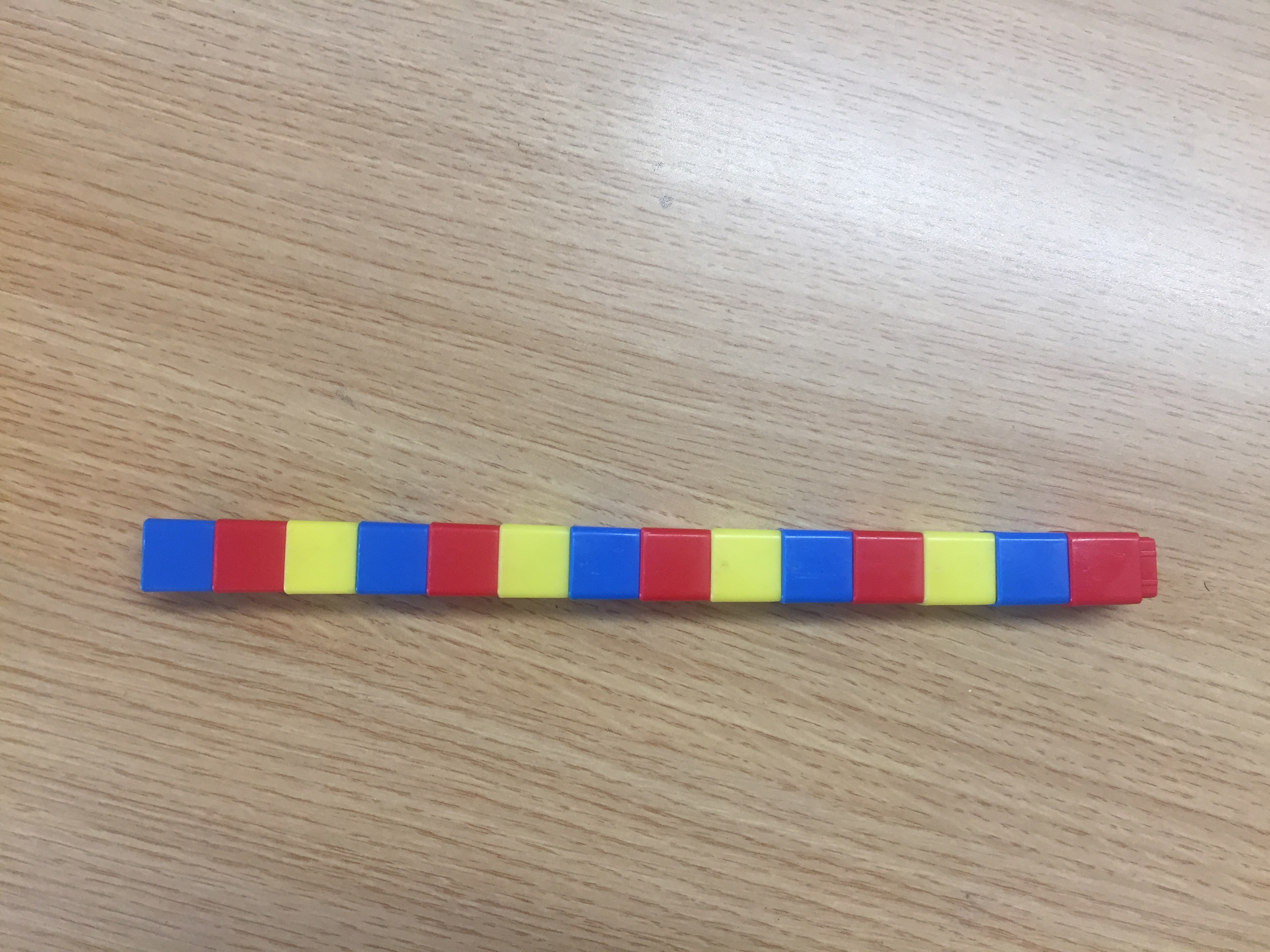### Sequences

Unlike repeating patterns, sequences are more linear; they tend to increase or decrease in specific ways; thus they are also referred to as increasing and decreasing patterns. The way in which the terms (the individual parts of the sequence) are ordered is governed by a rule. Similar to repeating patterns, the children need to be able to identify this rule in order to extend the sequence. To help the children identify the rule in numerical sequences, they should be encouraged to examine each given term and identify what has happened between it and the next term i.e. did the numbers increase, decrease, by how much etc.? They should then record this (e.g. +2, –3) below and between the terms, as modelled in the Operation Maths Pupil books and Discovery books.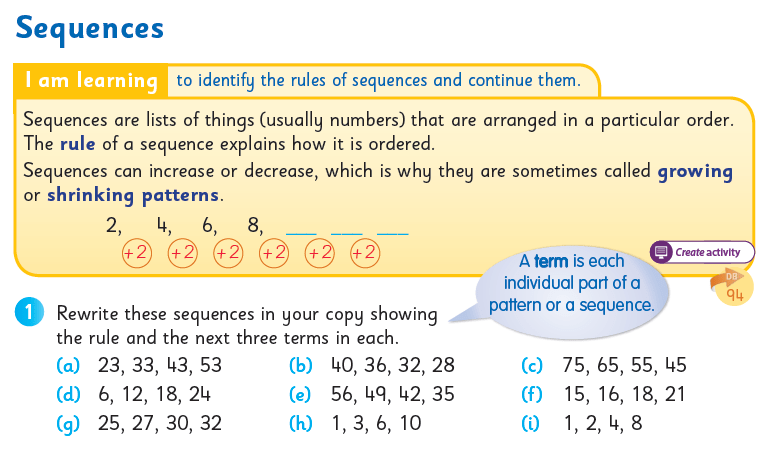Even when extending sequences in their copies, the children should be encouraged to leave an empty line below the sequences, to allow space for them to write in the differences between the terms.

Once the children are comfortable with sequences that increase/decrease by the same amount each term, they can progress to sequences that increase/decrease in varying but repetitive amounts, e.g. +2, +3, +2, …

### Odd and even numbers

Odd and even numbers are an example of an increasing pattern/sequence, as the difference between each term is +2. Most children will find it simple enough to recognise odd and even numbers; typically they will tell you that if a number ends in 2, 4, 6, 8 or zero, is it even and, if it does not, it is odd. It is one thing to identify odd and even numbers in this way, but it’s another thing to visualise the numbers and appreciate why they are odd or even. Using concrete materials or pictorial representations is vital for the children to really develop their number sense
and their appreciation of how odd and even numbers interact.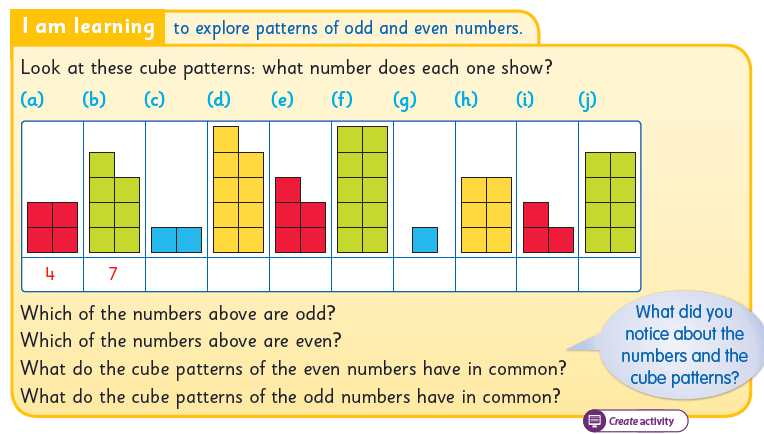As well as activities like that shown above, pairwise ten frames (ie ten frames placed vertically, as opposed to the more typical horizontal arrangement) can also be useful to model odd and even numbers. Such concrete or pictorial presentations can then be used to show how the total of an odd number and an odd number will always be even as the non-paired cube of both now join to form a pair. These activities also reinforce how only whole numbers can be classified as odd or even, even though a child may incorrectly assume that 1.2 is even, since it ends in 2.

### Identifying patterns in addition and multiplication facts

Through concrete activities and activities using the 100 square, it is hoped that the children begin to appreciate the patterns in number facts and that that various groups of multiples are characterised by certain properties eg.:

• When adding/subtracting 10 on the 100 square the answer is always the number directly below/above the starting number.
• When adding/subtracting 9 on the 100 square the answer is always the number diagonally below left /diagonally above right the starting number.
• The multiples of 10 always end in zero
• The digits in the multiples of 9 always total 9 or a multiple of 9 (e.g. 9 × 11 = 99 and 9 + 9 = 18), etc.

Not only will knowledge like this greatly aid their ability to identify and recall the basic number facts, but it will also improve their ability to check and identify errors in their own, and others’ work (e.g. ‘173 × 5 = 858 … hmm, that can’t be correct because multiples of 5 should always end in 5 or 0; I need to do that again’).

### Using T-charts to organise information

In the senior classes, when the rules governing the sequences are becoming more complex and less obvious, T-charts can be a very useful way to organise and present  the information. They can be particularly useful to help highlight the patterns and how these patterns are developing (see below). They can also provide the children with a clear way to explain how they see the rule. Therefore, the children should be encouraged to use them as a problem-solving strategy as much as is suitable.